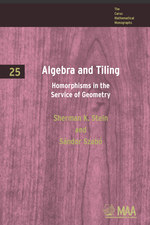# Algebra and Tiling: Homomorphisms in the Service of Geometry

Sherman K. Stein
Sándor Szabó
Volume: 25
Edition: 1
Pages: 222
https://www.jstor.org/stable/10.4169/j.ctt5hh7z6

1. Front Matter
(pp. i-vi)
2. Preface
(pp. vii-x)
(pp. xi-xii)
4. Chapter 1 Minkowski’s Conjecture
(pp. 1-34)

The origins of most of the tiling problems we will explore go back just a short time. But Minkowski’s problem, like many ideas in mathematics, can trace its roots to the Pythagorean theorem,\${a^2} + {b^2} = {c^2}\$. We shall trace these roots, and then go on to describe some of the problems that Minkowski’s question, in turn, suggested. We do this in order to place the problem in its proper position in the intricate web that constitutes mathematics.

The Greeks discovered all integer solutions of the equation\${x^2} + {y^2} = {z^2}\$pick three integersd,m, andn, and let one ofxandybe...

5. Chapter 2 Cubical Clusters
(pp. 35-56)

In Chapter 1 we were concerned with the way translates of a single cube fit together to tile space. In this chapter we examine tilings by translates of a finite collection of cubes, which we will call “clusters.” Chapters 3 and 4 will treat a special family of clusters that exists in all dimensions. Before we can state the main results of this chapter, we need some definitions.

As in Chapter 1, we assume a fixed coordinate system. We continue to identify each unit cube whose edges are parallel to the axes with its vertex that has the smallest coordinates....

6. Chapter 3 Tiling by the Semicross and Cross
(pp. 57-84)

When we hear the expression “convex body” we probably visualize such famous sets as the ball, the cube, or the tetrahedron. However, the expression “non-convex body” triggers no specific image, just the general sense of an object with dents. In this chapter and the next we explore two families of non-convex sets that may well be viewed as the prototypes of non-convex bodies.

These two particular families of clusters inn-space have drawn the attention of mathematicians for several reasons. First, their tiling, packing, and covering properties can be analyzed with the aid of existing algebraic and combinatorial tools. Second,...

7. Chapter 4 Packing and Covering by the Semicross and Cross
(pp. 85-106)

When a clusterCdoes not tilen-space, we immediately face two questions: How densely can we pack translates ofC? How thinly can we place translates ofCsuch that they cover all ofn-space? Similar questions for then-dimensional ball, going back to Kepler, have been answered only for small values ofn.We will get good estimates in all dimensions in the case ofZ-lattice packings by semi-crosses and even much more information about packing by crosses. Similar questions aboutZ-lattice coverings seem to be far more difficult, as we will see later in the chapter.

In...

8. Chapter 5 Tiling by Triangles of Equal Areas
(pp. 107-134)

In the first four chapters we examined tilings of Euclidean space by translates of sets that are unions of cubes. In this chapter we consider tilings of a much different character, namely tilings of polygons by triangles of equal areas. (We do not demand that the triangles be congruent.) We restrict our attention to simplicial dissections, though the theorems hold for all dissections.

In 1965 Fred Richman at the University of New Mexico at Las Cruces wanted to include a geometric question in a master’s degree examination he was preparing. “I noticed that it was easy to cut a square...

9. Chapter 6 Tiling by Similar Triangles
(pp. 135-154)

Lajos Pósa wrote his first paper when still in primary school and is well known for his work in graph theory. An enthusiastic teacher, he tries to convey the beauty of mathematics to students of all abilities, from the most talented to the least able, and of all ages, from small children to candidates for the doctorate. Often he holds irregular classes in the most remote towns.

He also organizes summer schools to which he invites students from all over Hungary. In 1987, when preparing for such a session he decided he needed a concrete geometry problem. Now, it is...

10. Chapter 7 Rédei’s Theorem
(pp. 155-186)

As a young mathematician, G. Hajós prepared a Ph.D. thesis on certain determinant identities. The chairman of his doctoral committee, L. Fejér, whose name is closely associated with Fourier analysis, feeling that the result did not match the outstanding talent of the candidate, rejected the thesis. This is why Hajós turned to Minkowski’s famous unsolved conjecture.

In 1938 Hajós formulated the problem in terms of factorizations of groups and, making use of this reformulation, refuted Furtwängler’s conjecture about multiple cube tilings, described in Chapter 1. This time his thesis met Fejér’s legendary high standards.

Almost everyone, on first meeting the...

11. Epilog
(pp. 187-188)

While the central theme of the seven chapters has been the interplay of geometry with algebra, another theme, purely algebraic, also ran through the book. Time and time again we have used mappings from one structure to another to simplify a problem.

In Chapters 1 and 2, where we faced an infinite latticeLimbedded in a rational latticeL', we formed the quotient groupL'/L.The natural homomorphism fromL'toL'/Lenabled us to work inL'/L,which is a finite group, instead of an infinite group.

At the end of Chapter 2 the solution of a brick-tiling...

12. Appendix A Lattices
(pp. 189-192)
13. Appendix B The Character Group and Exact Sequences
(pp. 193-196)
14. Appendix C Formal Sums
(pp. 197-198)
15. Appendix D Cyclotomic Polynomials
(pp. 199-200)
16. Bibliography for Preface
(pp. 201-202)
17. Supplement to the Bibliography
(pp. 203-204)
18. Name Index
(pp. 205-206)
19. Subject Index
(pp. 207-208)
20. Symbol Index
(pp. 209-209)